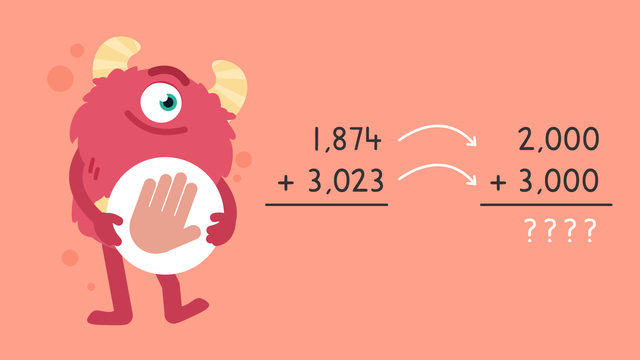# Estimating Sums and Differences— Let's PracticeRating

Be the first to give a rating!
The authorsTeam Digital
Estimating Sums and Differences— Let's Practice
CCSS.MATH.CONTENT.4.NBT.A.3

## Basics on the topicEstimating Sums and Differences— Let's Practice

Today we are practicing estimating sums and differences with Razzi! This video contains examples to help you further practice and grow confident in this area.

### TranscriptEstimating Sums and Differences— Let's Practice

Razzi says get these items ready because today we're going to practice estimating sums and differences. It's time to begin! Estimate the sum of one thousand eight hundred seventy-four and three thousand twenty-three. Pause the video to work on the problem and press play when you are ready to see the solution! One thousand eight hundred seventy-four rounds to two thousand and three thousand twenty-three rounds to three thousand. Did you also get the estimated sum of five thousand? Let's tackle the next problem! Estimate the sum of five thousand one hundred thirty-five and four thousand eight hundred thirty-two. Pause the video to work on the problem and press play when you are ready to see the solution! Five thousand one hundred thirty-five rounds to five thousand and four thousand eight hundred thirty-two also rounds five thousand. Did you also get the estimated sum of ten thousand? Let's tackle the final problem! Estimate the difference of nine thousand three hundred sixty-three and two thousand six hundred twelve. Pause the video to work on the problem and press play when you are ready to see the solution! Nine thousand three hundred sixty-three rounds to nine thousand... and two thousand six hundred twelve rounds to three thousand. Did you also get the estimated difference of six thousand? Razzi had so much fun practicing with you today! See you next time!

## Estimating Sums and Differences— Let's Practice exercise

Would you like to apply the knowledge you’ve learned? You can review and practice it with the tasks for the video Estimating Sums and Differences— Let's Practice .
• ### Place the estimates where they belong.

Hints

Remember, when we round numbers, we round to the nearest place value. For example, 7,872 would round to 8,000. 7,872 is closer to 8,000 than 7,000.

Look at the number by place value. For example, 5,098 would round to 5,000, since there is a zero in the hundreds place. That makes 5,098 closer to 5,000 than 6,000.

Solution

6,712 rounds up to 7,000

3,298 rounds down to 3,000

1,900 rounds up to 2,000

1,004 rounds down to 1,000

• ### Estimate the digits and the sum to the nearest 1,000.

Hints

Remember, when we estimate, we round a digit up or down based on the place value. For example, 5,930 would round to 6,000, because it is closer to 6,000 than 5,000.

7,324 would round to 7,000, since it is closer to 7,000 than 8,000.

Add the two estimates together to find the estimated sum.

Solution

4,071 rounds down to 4,000, and 4,913 rounds up to 5,000. Now we can estimate the sum: 4,000 + 5,000 = 9,000

• ### Fill in the missing numbers.

Hints

When we round a number, we look at the place value of each digit.

For example, look at the number 5,321. We know this number starts with 5,000. The next digit is in the hundreds place, 300. Since 300 is lower than 500, the number is rounded down. 5,321 rounds to 5,000.

If you are unsure of rounding up or down, look at the hundreds place. Then, look at the tens place. Finally, look at the ones place.

Remember, the estimates should add up to 11,000.

Solution

6,646 rounds up to 7,000. 3,711 rounds up to 4,000.

7,000 + 4,000 = 11,000.

• ### Fill in the missing estimates.

Hints

Remember, when we estimate we round the digit to the nearest thousand.

For example, 6,786 would round to 7,000, because it is closer than 6,000.

To find the total estimate, subtract the second estimate from the first.

If you are unsure of rounding up or down, look at the hundreds place. Then, look at the tens place. Finally, look at the ones place.

Solution

8,598 rounds up to 9,000. 5,523 rounds up to 6,000.

9,000 - 6,000 = 3,000

• ### Estimate the sum.

Hints

Remember to round each number to estimate the sum.

Look at the number 3,754. Is it closer to 3,000, or to 4,000?

Look at the number 4,213. Is it closer to 5,000, or 4,000?

Solution

We round 3,754 up to 4,000. Then, we round 4,213 down to 4,000.

4,000 + 4,000 = 8,000

• ### Fill in the difference.

Hints

Remember, first round each digit to the nearest thousand. Then, estimate the difference.

Look at the number 4,798. Look at the hundreds place: 4,798. Since 700 is closer to 1,000, we round this number up to the next thousand. 4,798 rounds to 5,000.

Solution

12,421 rounds down to 12,000.

6,882 rounds up to 7,000.

12,000 - 7,000 = 5,000